# Layouts

#### 2021-02-22

In very short terms, a layout is the vertical and horizontal placement of nodes when plotting a particular graph structure. Conversely, a layout algorithm is an algorithm that takes in a graph structure (and potentially some additional parameters) and return the vertical and horizontal position of the nodes. Often, when people think of network visualizations, they think of node-edge diagrams where strongly connected nodes are attempted to be plotted in close proximity. Layouts can be a lot of other things too though — e.g. hive plots and treemaps. One of the driving factors behind `ggraph` has been to develop an API where any type of visual representation of graph structures is supported. In order to achieve this we first need a flexible way of defining the layout…

## The ggraph() and create_layout() functions

As the layout is a global specification of the spatial position of the nodes it spans all layers in the plot and should thus be defined outside of calls to geoms or stats. In `ggraph` it is often done as part of the plot initialization using `ggraph()` — a function equivalent in intent to `ggplot()`. As a minimum `ggraph()` must be passed a graph object supported by `ggraph`:

``````library(ggraph)
library(tidygraph)

set_graph_style(plot_margin = margin(1,1,1,1))
graph <- as_tbl_graph(highschool)

# Not specifying the layout - defaults to "auto"
ggraph(graph) +
geom_node_point()``````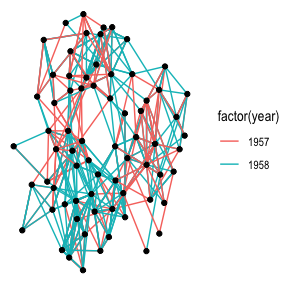Not specifying a layout will make `ggraph` pick one for you. This is only intended to get quickly up and running. The choice of layout should be deliberate on the part of the user as it will have a great effect on what the end result will communicate. From now on all calls to `ggraph()` will contain a specification of the layout:

``````ggraph(graph, layout = 'kk') +
geom_node_point()``````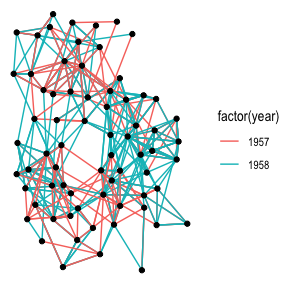If the layout algorithm accepts additional parameters (most do), they can be supplied in the call to `ggraph()` as well:

``````ggraph(graph, layout = 'kk', maxiter = 100) +
geom_node_point()``````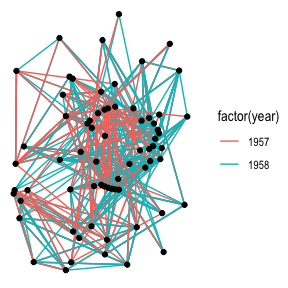If any layout parameters refers to node or edge variables they must be supplied as unquoted expression (like inside `aes()` and `tidyverse` verbs)

In addition to specifying the layout during plot creation it can also happen separately using `create_layout()`. This function takes the same arguments as `ggraph()` but returns a `layout_ggraph` object that can later be used in place of a graph structure in ggraph call:

``layout <- create_layout(graph, layout = 'eigen')``
``````## Warning in layout_with_eigen(graph, type = type, ev = eigenvector): g is
## directed. undirected version is used for the layout.``````
``````ggraph(layout) +
geom_node_point()``````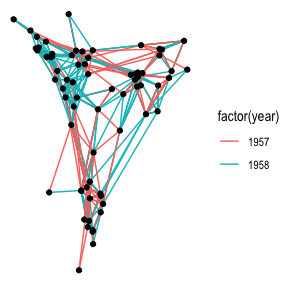Examining the return of `create_layout()` we see that it is really just a `data.frame` of node positions and (possible) attributes. Furthermore the original graph object along with other relevant information is passed along as attributes:

``head(layout)``
``````##             x           y circular name .ggraph.orig_index .ggraph.index
## 1 -0.04317633 -0.15611549    FALSE    1                  1             1
## 2 -0.03414457 -0.20843892    FALSE    2                  2             2
## 3 -0.04963286 -0.30081095    FALSE    3                  3             3
## 4  0.18211675  0.03597610    FALSE    4                  4             4
## 5  0.18048193 -0.01111635    FALSE    5                  5             5
## 6  0.01389960 -0.19521616    FALSE    6                  6             6``````
``attributes(layout)``
``````## \$names
##  "x"                  "y"                  "circular"
##  "name"               ".ggraph.orig_index" ".ggraph.index"
##
## \$row.names
##    1  2  3  4  5  6  7  8  9 10 11 12 13 14 15 16 17 18 19 20 21 22 23 24 25
##  26 27 28 29 30 31 32 33 34 35 36 37 38 39 40 41 42 43 44 45 46 47 48 49 50
##  51 52 53 54 55 56 57 58 59 60 61 62 63 64 65 66 67 68 69 70
##
## \$class
##  "layout_tbl_graph" "layout_ggraph"    "data.frame"
##
## \$graph
## # A tbl_graph: 70 nodes and 506 edges
## #
## # A directed multigraph with 1 component
## #
## # Node Data: 70 x 2 (active)
##   name  .ggraph.orig_index
##   <chr>              <int>
## 1 1                      1
## 2 2                      2
## 3 3                      3
## 4 4                      4
## 5 5                      5
## 6 6                      6
## # … with 64 more rows
## #
## # Edge Data: 506 x 3
##    from    to  year
##   <int> <int> <dbl>
## 1     1    13  1957
## 2     1    14  1957
## 3     1    20  1957
## # … with 503 more rows
##
## \$circular
##  FALSE``````

As it is just a `data.frame` it means that any standard `ggplot2` call will work by addressing the nodes. Still, use of the `geom_node_*()` family provided by `ggraph` is encouraged as it makes it explicit which part of the data structure is being worked with.

## Adding support for new data sources

Out of the box `ggraph` supports `tbl_graph` objects from tidygraph natively. Any other type of object will be attempted to be coerced to a `tbl_graph` object automatically. Tidygraph provide conversions for most known graph structure in R so almost any data type is supported by ggraph by extension. If there is wish for support for additional classes this can be achieved by providing a `as_tbl_graph()` method for the class. If you do this, consider submitting the method to tidygraph so others can benefit from your work.

## Layouts abound

There’s a lot of different layouts in `ggraph` — All layouts from the graphlayouts and igraph packages are available, an ggraph itself also provide some of the more specialised layouts itself. All in all ggraph provides well above 20 different layouts to choose from, far more than we can cover in this text. I urge you to explore the different layout types. Blindly running along with the default layouts is a sad but common mistake in network visualisation that can cloud or distort the insight the network might hold. If ggraph lacks the needed layout it is always possible to supply your own layout function that takes a tbl_graph object and returns a data.frame of node positions, or supply the positions directly by passing a matrix or data.frame to the layout argument.

### A note on circularity

Some layouts can be shown effectively both in a standard Cartesian projection as well as in a polar projection. The standard approach in `ggplot2` has been to change the coordinate system with the addition of e.g. `coord_polar()`. This approach — while consistent with the grammar — is not optimal for `ggraph` as it does not allow layers to decide how to respond to circularity. The prime example of this is trying to draw straight lines in a plot using `coord_polar()`. Instead circularity is part of the layout specification and gets communicated to the layers with the `circular` column in the data, allowing each layer to respond appropriately. Sometimes standard and circular representations of the same layout get used so often that they get different names. In `ggraph` they’ll have the same name and only differ in whether or not `circular` is set to `TRUE`:

``````# An arc diagram
ggraph(graph, layout = 'linear') +
geom_edge_arc(aes(colour = factor(year)))``````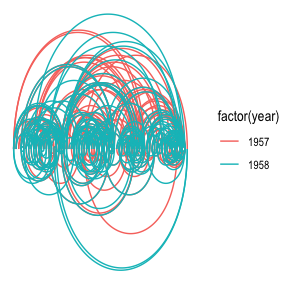``````# A coord diagram
ggraph(graph, layout = 'linear', circular = TRUE) +
geom_edge_arc(aes(colour = factor(year))) +
coord_fixed()``````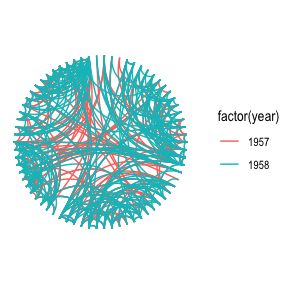``````graph <- tbl_graph(flare\$vertices, flare\$edges)
# An icicle plot
ggraph(graph, 'partition') +
geom_node_tile(aes(fill = depth), size = 0.25)``````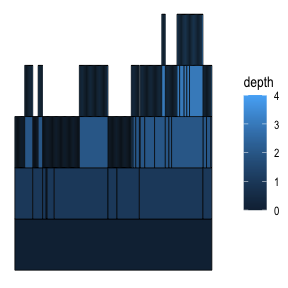``````# A sunburst plot
ggraph(graph, 'partition', circular = TRUE) +
geom_node_arc_bar(aes(fill = depth), size = 0.25) +
coord_fixed()``````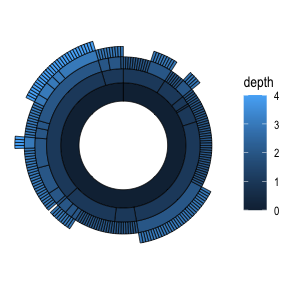Not every layout has a meaningful circular representation in which cases the `circular` argument will be ignored.

### Node-edge diagram layouts

Both `graphlayout` and `igraph` provides a range of different layout algorithms for classic node-edge diagrams (colloquially referred to as hairballs). Some of these are incredibly simple such as randomly, grid, circle, and star, while others tries to optimize the position of nodes based on different characteristics of the graph. There is no such thing as “the best layout algorithm” as algorithms have been optimized for different scenarios. Experiment with the choices at hand and remember to take the end result with a grain of salt, as it is just one of a range of possible “optimal node position” results. Below is a sample of some of the layouts available through `igraph` applied to the highschool graph.

``````graph <- as_tbl_graph(highschool) %>%
mutate(degree = centrality_degree())
lapply(c('stress', 'fr', 'lgl', 'graphopt'), function(layout) {
ggraph(graph, layout = layout) +
geom_edge_link(aes(colour = factor(year)), show.legend = FALSE) +
geom_node_point() +
labs(caption = paste0('Layout: ', layout))
})``````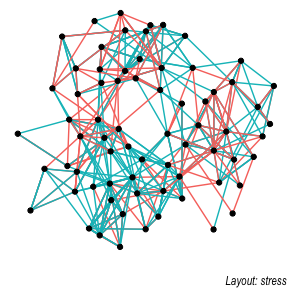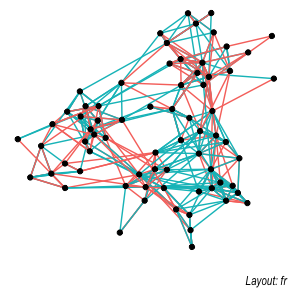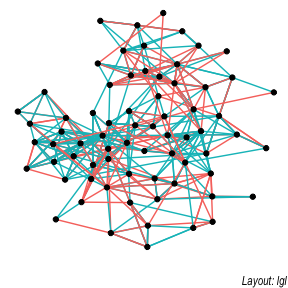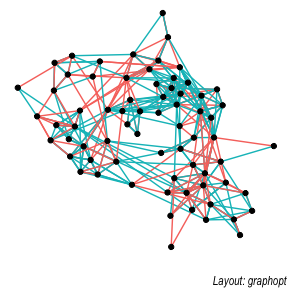### Hive plots

A hive plot, while still technically a node-edge diagram, is a bit different from the rest as it uses information pertaining to the nodes, rather than the connection information in the graph. This means that hive plots, to a certain extent are more interpretable as well as less vulnerable to small changes in the graph structure. They are less common though, so use will often require some additional explanation.

``````graph <- graph %>%
mutate(friends = ifelse(
centrality_degree(mode = 'in') < 5, 'few',
ifelse(centrality_degree(mode = 'in') >= 15, 'many', 'medium')
))
ggraph(graph, 'hive', axis = friends, sort.by = degree) +
geom_edge_hive(aes(colour = factor(year))) +
geom_axis_hive(aes(colour = friends), size = 2, label = FALSE) +
coord_fixed()``````